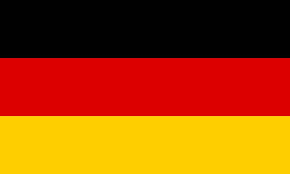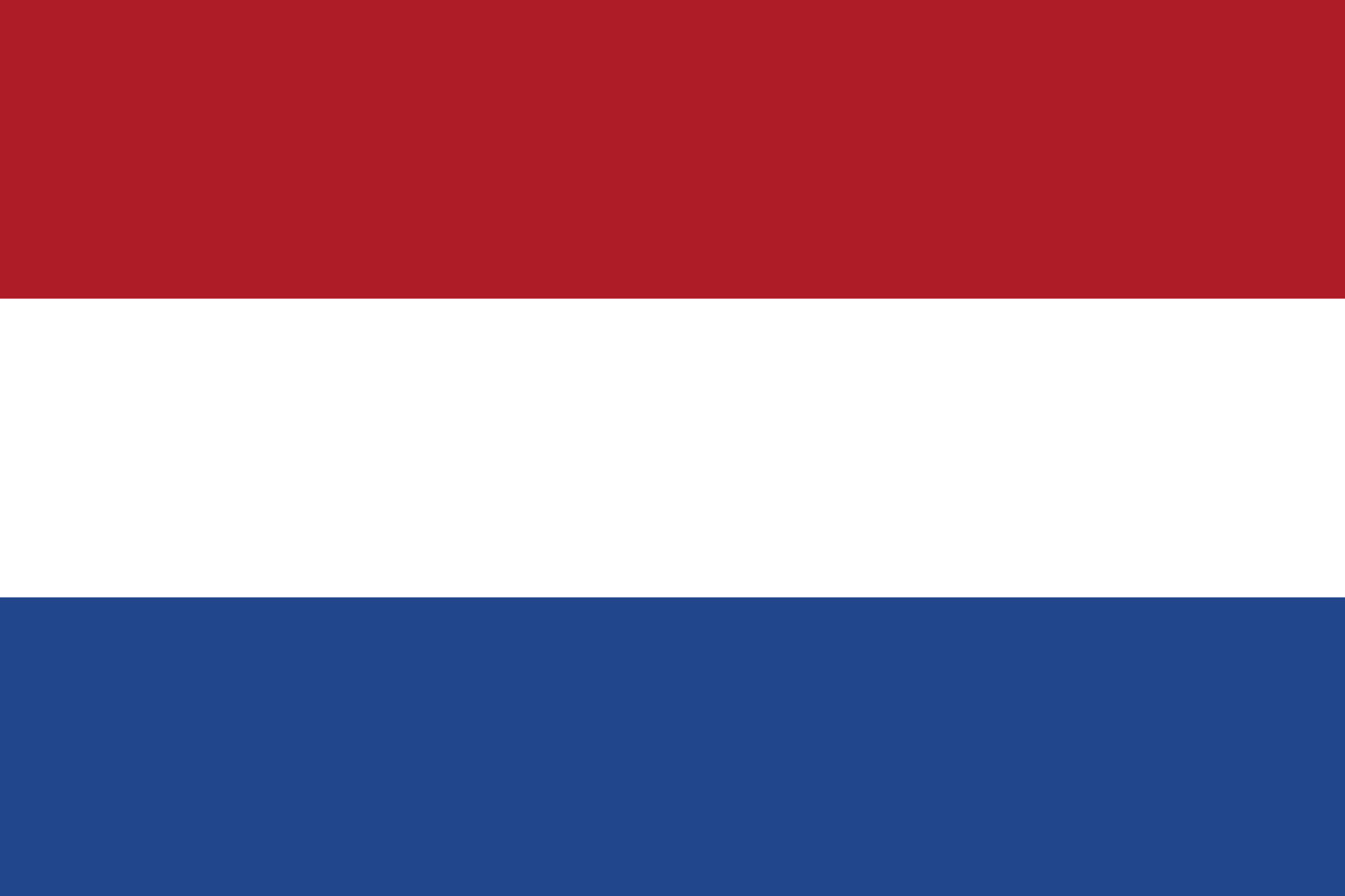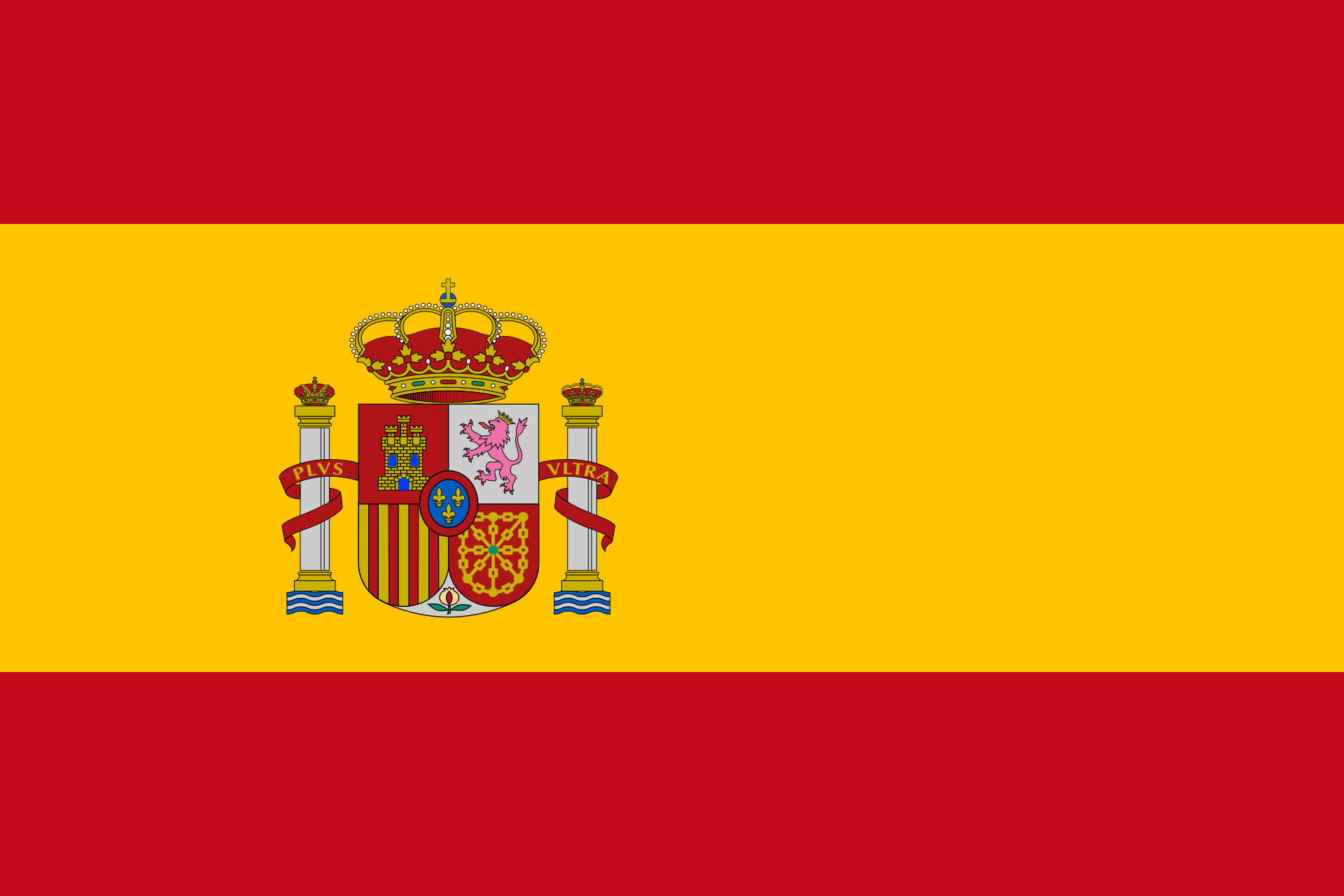# Intersection line planeChoose how the line is given.
• parametric equation:

 g: x =+ r• Given through two points

Intersection with plane

Choose how the plane is given.
• parametric equation:

 E: x =+ r+ s• Coordinate form:

• Point-normal form:

 E: (x-)⋅=0
• Given through three points